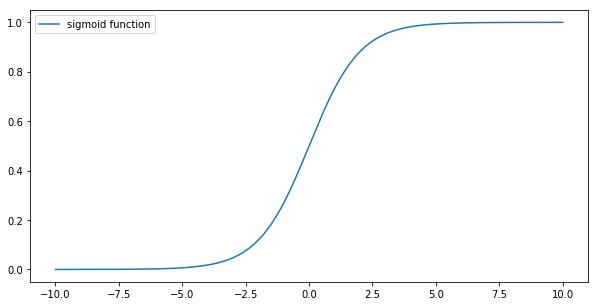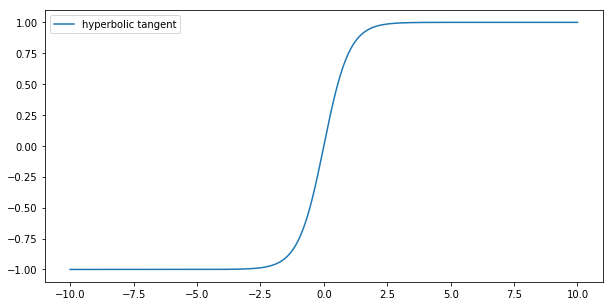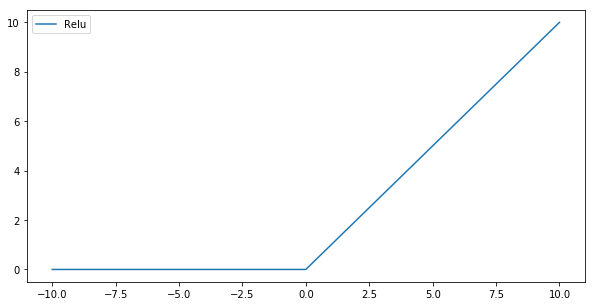In :
import numpy as np
import matplotlib.pyplot as plt
%matplotlib inline


#### Sigmoid $\sigma{(x)}$¶

$$\sigma{(x)} = \frac{1}{1 + e^{-x}}$$

Function flattens rather quickly (values go to 0 or 1). This is causing partial derivatives going to zero quickly as well, as a result the weigths cannot be updated and the model cannot learn. This can be mitigated by proper weigth inicialization.

In :
x = np.linspace(-10, 10, 1000)
y = 1 / (1 + np.exp(-x) )

plt.figure(figsize=(10, 5))
plt.plot(x, y)
plt.legend(['sigmoid function'])
plt.show()#### Tanh (hyperbolic tangent)¶

$$\tanh{(x)} = \frac{e^{x}-e^{-x}}{e^{x}+e^{-x}} = 2 \sigma{(2x)} - 1$$

Tanh can be thought of as a scaled sigmoid and has similar issues with gradients as the original sigmoid function. Adjusting weights will supress model issues with vanishing gradients.

In :
x = np.linspace(-10, 10, 1000)
y = ( 2 / (1 + np.exp(-2*x) ) ) -1

plt.figure(figsize=(10, 5))
plt.plot(x, y)
plt.legend(['hyperbolic tangent'])
plt.show()#### ReLU¶

Rectified Linear Unit

$$f(x) = max(0, x)$$

ReLU functions help to achieve fast convergence, so the model trains quickly. It is also faster to compute derivatives on essentially linear function.

The issue is with the constant part of the function (where $f(x) = 0$), this is where gradients are zero as well. Gradient descent process cannot continue and training of the model goes to a halt.

Learning rate tweaking will mitigate this issue.

In :
x = np.linspace(-10, 10, 1000)
y = np.maximum(0, x)

plt.figure(figsize=(10, 5))
plt.plot(x, y)
plt.legend(['Relu'])
plt.show()